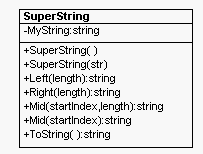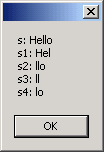Today I realized that I miss those Visual Basic/Visual C++ type operators. You know the ones:  Left, Mid, Right.  I'm so used to using them, that I decided to override the string class.  Simple enough, right?  I just inherit from the System.String and then I get all the nice string methods, plus my own: Left, Mid, and Right.  The problem, though, is that the System.String class is sealed.  You can't inherit from it. So now what?  Must I give up the dream of using those fine old methods?  Obviously you can do everything with Substring that you can with Left, Right and Mid.  But I'm stubborn so I turned to another solution, aggregation.  If you can't use inheritance, then you can simply embed the string inside your new class as a private member and utilize it internally.  The design for the class is shown below:Fig 1.  Class design created using WithClass 2000

To utilize functions of the SuperString class simply call:

MySuperString.TheSuperStringFunction();

If you want to utilize the string functions of the string class simply call:

MySuperString.ToString().TheStringFunction();

Below is the code for the Visual Basic Functions Mid, Left, and Right. Note that we had to use Substring to impliment them:

public string Left(int length)
{
string tmpstr = MyString.Substring(0, length);
return tmpstr;
}
public string Right(int length)
{
string tmpstr = MyString.Substring(MyString.Length - length, length);
return tmpstr;
}
public string Mid(int startIndex, int length)
{
string tmpstr = MyString.Substring(startIndex, length);
return tmpstr;
}
public string Mid(int startIndex)
{
string tmpstr = MyString.Substring(startIndex);
return tmpstr;
}

Listing 1 - The SuperString functions Mid, Left and Right.

Below is the code in our form that shows how we utilized these functions and the corresponding output:

SuperString s = "Hello";
string s1 = s.Left(3);
string s2 = s.Right(3);
string s3 = s.Mid(2,2);
string s4 = s.Mid(3);
MessageBox.Show( "s: " + s + "\n" +"s1: " + s1 + "\n" +"s2: " + s2 + "\n" +"s3: " + s3 + "\n" +"s4: " + s4 + "\n");Fig 2 - Output for SuperString Example

Also worth noting are two more functions added to the string class to allow for implicit and explicit conversion back and forth between string and SuperString.  Below are the static functions:

// override the ToString function to return the SuperString as a string
public override string ToString()
{
return MyString; // return the internal string
}
// Implicit Conversion from string to SuperString
// creates a new SuperString and returns it
public static implicit operator SuperString(string x)
{
SuperString s =
new SuperString(x);
return s;
}
// Explicit conversion from SuperString to string. returns
// the internal string
public static explicit operator string(SuperString x)
{
return x.MyString;
}